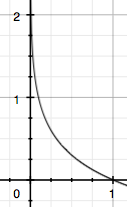# 关于交叉熵在loss函数中使用的理解

## 信息论

### 1 信息量

I(x0)=log(p(x0)) I ( x 0 ) = − l o g ( p ( x 0 ) )### 2 熵

A电脑正常开机0.7-log(p(A))=0.36
B电脑无法开机0.2-log(p(B))=1.61
C电脑爆炸了0.1-log(p(C))=2.30

H(X)=i=1np(xi)log(p(xi)) H ( X ) = − ∑ i = 1 n p ( x i ) l o g ( p ( x i ) )

H(X)===[p(A)log(p(A))+p(B)log(p(B))+p(C))log(p(C))]0.7×0.36+0.2×1.61+0.1×2.300.804(1)(2)(3) (1) H ( X ) = − [ p ( A ) l o g ( p ( A ) ) + p ( B ) l o g ( p ( B ) ) + p ( C ) ) l o g ( p ( C ) ) ] (2) = 0.7 × 0.36 + 0.2 × 1.61 + 0.1 × 2.30 (3) = 0.804

H(X)==i=1np(xi)log(p(xi))p(x)log(p(x))(1p(x))log(1p(x))(4)(5) (4) H ( X ) = − ∑ i = 1 n p ( x i ) l o g ( p ( x i ) ) (5) = − p ( x ) l o g ( p ( x ) ) − ( 1 − p ( x ) ) l o g ( 1 − p ( x ) )

### 3 相对熵（KL散度）

In the context of machine learning, DKL(P‖Q) is often called the information gain achieved if P is used instead of Q.

KL散度的计算公式：

DKL(p||q)=i=1np(xi)log(p(xi)q(xi))(3.1) (3.1) D K L ( p | | q ) = ∑ i = 1 n p ( x i ) l o g ( p ( x i ) q ( x i ) )

n为事件的所有可能性。
DKL D K L $D_{KL}$的值越小，表示q分布和p分布越接近

### 4 交叉熵

DKL(p||q)==i=1np(xi)log(p(xi))i=1np(xi)log(q(xi))H(p(x))+[i=1np(xi)log(q(xi))](6)(7) (6) D K L ( p | | q ) = ∑ i = 1 n p ( x i ) l o g ( p ( x i ) ) − ∑ i = 1 n p ( x i ) l o g ( q ( x i ) ) (7) = − H ( p ( x ) ) + [ − ∑ i = 1 n p ( x i ) l o g ( q ( x i ) ) ]

H(p,q)=i=1np(xi)log(q(xi)) H ( p , q ) = − ∑ i = 1 n p ( x i ) l o g ( q ( x i ) )

## 机器学习中交叉熵的应用

### 1 为什么要用交叉熵做loss函数？

loss=12mi=1m(yiyi^)2 l o s s = 1 2 m ∑ i = 1 m ( y i − y i ^ ) 2

MSE在线性回归问题中比较好用，那么在逻辑分类问题中还是如此么？

### 2 交叉熵在单分类问题中的使用

loss=i=1nyilog(yi^)(2.1) (2.1) l o s s = − ∑ i = 1 n y i l o g ( y i ^ )*青蛙老鼠
Label010
Pred0.30.60.1

loss==(0×log(0.3)+1×log(0.6)+0×log(0.1)log(0.6)(8)(9) (8) l o s s = − ( 0 × l o g ( 0.3 ) + 1 × l o g ( 0.6 ) + 0 × l o g ( 0.1 ) (9) = − l o g ( 0.6 )

loss=1mj=1mi=1nyjilog(yji^) l o s s = − 1 m ∑ j = 1 m ∑ i = 1 n y j i l o g ( y j i ^ )

m为当前batch的样本数

### 3 交叉熵在多分类问题中的使用*青蛙老鼠
Label011
Pred0.10.70.8

loss=ylog(ŷ )(1y)log(1ŷ ) l o s s = − y l o g ( y ^ ) − ( 1 − y ) l o g ( 1 − y ^ )

losslossloss===0×log(0.1)(10)log(10.1)=log(0.9)1×log(0.7)(11)log(10.7)=log(0.7)1×log(0.8)(11)log(10.8)=log(0.8)(10)(11)(12) (10) l o s s 猫 = − 0 × l o g ( 0.1 ) − ( 1 − 0 ) l o g ( 1 − 0.1 ) = − l o g ( 0.9 ) (11) l o s s 蛙 = − 1 × l o g ( 0.7 ) − ( 1 − 1 ) l o g ( 1 − 0.7 ) = − l o g ( 0.7 ) (12) l o s s 鼠 = − 1 × l o g ( 0.8 ) − ( 1 − 1 ) l o g ( 1 − 0.8 ) = − l o g ( 0.8 )

loss=j=1mi=1nyjilog(yji^)(1yji)log(1yji^) l o s s = ∑ j = 1 m ∑ i = 1 n − y j i l o g ( y j i ^ ) − ( 1 − y j i ) l o g ( 1 − y j i ^ )

## 总结

04-253万+06-10770
10-251万+
07-2379
03-0314万+
06-241337
09-142万+
06-252727
01-215055
©️2020 CSDN 皮肤主题: 编程工作室 设计师:CSDN官方博客